# Monarchs and Matched Filtering¶

In :
import numpy
import matplotlib.pyplot as plt
from scipy.interpolate import InterpolatedUnivariateSpline
from scipy.integrate import odeint
from IPython.display import Latex
from IPython.display import Image
import binascii
from numpy.linalg import pinv
import random
import scipy
%matplotlib inline


## A Monarch in ISM Band¶

The Monarch transmits at 915 MHz, at a wavelength of approximately 32.8 cm. Let us consider the case of two antennas (transmit and receive antennas) in free space separated by a distance $R$.

#### Derivation of Friis Equation¶

Let us assume that $P_T$ Watts of total power is delivered to the transmit antenna, which (for the moment) is assumed to be omnidirectional and lossless. Furthermore, we'll assume that the receive antenna is in the far field of the the transmit antenna (a safe assumption for transmissions from orbit). As the signal propagates spherically out from the transmit antenna, the power density (watts per square meter) of the plane wave decreases. By the time the signal reaches the receive antenna at a distance $R$ away, the power density is given by:

\begin{align} p &= \frac{P_T}{4\pi R^2} \end{align}

Any losses and directionality of the transmit antenna can be absorbed by a gain $G_T$. A transmit gain greater than one for a lossless antenna means that it is transmitting in a preferrred direction, and that direction is towards the receive antenna. Augmenting the above equation:

\begin{align} p &= \frac{P_T}{4\pi R^2} G_T \end{align}

Now consider the receive antenna. The aperature (i.e. effective area or receiving cross section) of an antenna is a measure of how effective an antenna is at receiving the power of radio waves. It is the area, oriented perpendicular to the direction of an incoming radio wave, that would intercept the same amount of power from that wave as is produced by the antenna receiving it. We can therefore augment the equation again to get received power:

\begin{align} P_R &= \frac{P_T}{4\pi R^2}G_T A_{ER} \end{align}

The aperature for any antenna can also be expressed as:

\begin{align} A_{ER} &= \frac{\lambda ^2}{4\pi}G_R \end{align}

(derivation based on thought experiment involving radiating resistive load in thermal equilibrium with an antenna, haven't grok'd this yet)

Rewriting again:

\begin{align} P_R &= \frac{P_TG_TG_R\lambda^2}{\left(4\pi R\right)^2} \end{align}

This is the Friis Transmission Formula. In the above equation, power is measured in linear units (watts). If, however, we convert to logarithmic units (decibels), we get the following:

\begin{align} 10\text{log}_{10}P_R = 10\text{log}_{10}\left(\frac{P_TG_TG_R\lambda^2}{\left(4\pi R\right)^2}\right) \end{align}
In :
def todBw(mW):
return 10*numpy.log10(mW) - 30

def todBm(mW):

def toMilliWatts(dBm):
return 10.**((dBm)/10.)

In :
todBw(25)

Out:
-16.020599913279625

Or, in decibels:

\begin{align} \boxed{[P_R]_{db} = [P_T]_{db} + [G_T]_{db} + [G_R]_{dB} + 10\text{log}_{10}\left[\left(\frac{\lambda}{4\pi R}\right)^{2}\right]} \end{align}

#### Reflecting on the Friis Equation¶

From the above equation, it can be seen that path loss increases as wavelength decreases (or as frequency increases). This is why cell phones generally operate at less than 2 GHz. The received power depends on the power of the transmitter, the gain of the transmitter, the gain of the receiver, the wavelength being used, and the distance between antennae. Let us now substitute in some realistic values for the Sprite.

The Monarch has a $25\text{mW} = -16 dBW$, isotropic ($G_T=0$) transmitter that communicates on the 32.8 cm ($\lambda=0.328$) band from orbit ($R=500000m$). On the receiving end, we'll assume a handheld yagi with a gain of approximately $G_R=7 dB$. Substituting and solving, we find:

In :
Pt = 25     #mW
Gt = 0.     #dBm (isotropic)
lam = 0.328   #meters
R = 500000. #meters
Gr = 7      #dBm


Coding up the Friis Equation:

In :
def friisEquation(Pt=Pt, Gt=Gt, Gc=0, Gr=Gr, lam=lam, R=R):
friisEquation()

Out:
-124.66672040620836
\begin{align} \boxed{[P_R]_{dbm} \approx -125} \end{align}

The noise power is given by the sum of the noise temperature and feed losses. The black body thermal noise is radiated from the environment, and the feed losses come from within the system.

\begin{align} P_n &= F_N + 10\text{log}_{10}\left(K_BTB\right) \end{align}

$K_B$ is the Botzmann constant, $T=150K$ is the background temperature, and $B=64 kHz$ is the receiver bandwidth. $F_N$ is the receiver noise figure, assumed to be $9dBW$ (coming largely from the low noise amplifier). Solving:

In :
Fn = 39.             #dBm
T = 150.             #Kelvin
B = 50000.           #Bandwidth (Hz)
Kb = 1.38064852e-23  #Boltzmann (SI Units)


Code up the noise equation:

In :
def noiseEquation(Fn=Fn, T=T, B=B, Kb=Kb):
return Fn + todBm(Kb*T*B)
noiseEquation()

Out:
-120.84855604918036
\begin{align} \boxed{P_{n} \approx -121 dB} \end{align}

This gives a signal to noise ratio (SNR) of approximately:

In :
def getSNRdBm(Gc=0):
return friisEquation(Gc=Gc) - noiseEquation()
getSNRdBm()

Out:
-3.818164357027996
\begin{align} \boxed{SNR = P_R - P_n = -3.8dB} \end{align}

If we were to represent received power and noise power in watts and find their ratio, the above result means that the ratio would be:

In :
def getSNRRatio(Gc=0):
getSNRRatio()

Out:
0.41512946933280453
\begin{align} \boxed{\frac{P_R}{P_n} =SNR \approx 10^{\frac{-3.8dB}{10}}= 0.415} \end{align}

#### Just how bad is this?¶

Consider the following signal, which varies in amplitude between -1 and 1:

Create a signal of the designated length:

In :
def createSignal(totransmit):
totransmit=str(totransmit)
test= [ord(c) for c in totransmit]
test1 = []
for i in test:
test1.extend(['{0:08b}'.format(i)])
test2 = ''
for i in test1:
test2 += i
data = ' '
for i in test2:
data+=i+' '
signal= numpy.fromstring(data, sep=' ')
return (2*signal-1)

In :
signal = createSignal('Greetings Earthlings')


Based on the variance of that signal and the signal to noise ratio, find the standard deviation of the additive Gaussian noise:

In :
def getNoiseSTD(signal, Gc=0):
return numpy.sqrt(1./getSNRRatio(Gc=Gc))


Generate a noise vector by drawing from a Gaussian with the appropriate standard deviation:

In :
def makeNoise(noiseSTD, signal):
return noiseSTD*numpy.random.randn(len(signal))


Create a function that compares the transmitted to the received signal:

In :
def runMonteCarlo(runs=100, ylim=4.5, Gc=0):
noiseSTD = getNoiseSTD(signal, Gc=Gc)
for i in range(runs):
noise = makeNoise(noiseSTD, signal)
plt.plot(signal+noise, alpha=0.1)
plt.plot(signal, 'r',lw=2, label='Signal', alpha=1)
plt.legend(loc='best')
plt.ylim([-ylim,ylim])
plt.show()


With all noise in view:

In :
runMonteCarlo()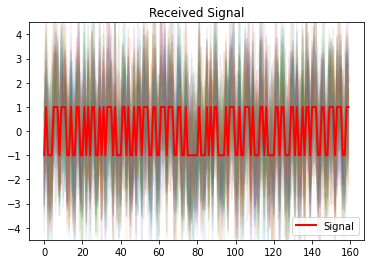Within a tighter range:

In :
runMonteCarlo(ylim=1.5)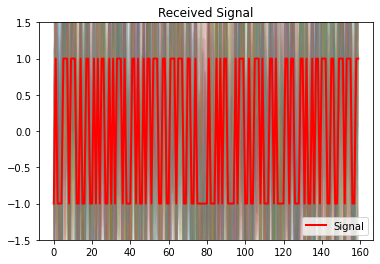#### What's the Bit Error Rate?¶

From the above SNR, we can solve for the variance of the additive Gaussian noise:

\begin{align} \sigma^2_{N} &= \frac{P_R}{SNR}\\ &\approx \frac{1 \text{ (assume received power is 1 in some unit)}}{0.415}\\ &\approx 2.4 \end{align}
In :
thresh=0.5

In :
def drawHistos(draws=1000, Gc=0):
plt.hist(getNoiseSTD(signal, Gc=Gc)*numpy.random.randn(draws)-1, bins=50,
alpha=0.5, label='p(-1)')
plt.hist(getNoiseSTD(signal, Gc=Gc)*numpy.random.randn(draws)+1, bins=50,
alpha=0.5, label='p( 1)')
plt.plot(numpy.ones(70)-thresh, numpy.arange(0,70,1), 'b--', label='threshold', lw=2)
plt.plot(numpy.ones(70)-(1+thresh), numpy.arange(0,70,1), 'g--', lw=2)
plt.legend(loc='best')
plt.show()
drawHistos(Gc=0)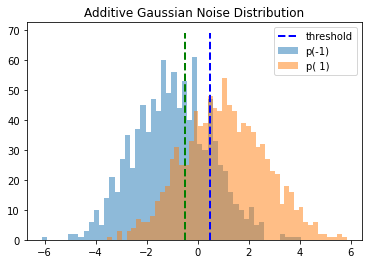Let us assume a thresholding of 0.5. That is to say, a signal above 0.5 is considered a 1, and a signal below -0.5 is considered a -1. These thresholds are plotted above. Let us furthermore assume that there is an equal probability of a 1 or a -1 being transmitted. The probability of a bit error is then given by:

\begin{align} p(error) &= p\left(\text{transmit 0}\right)\cdot p\left(\text{receive 1 }|\text{ transmit 0}\right) + p\left(\text{transmit 1}\right)\cdot p\left(\text{receive 0 }|\text{ transmit 1}\right) \end{align}
In :
PATH = "./"
Image(filename = PATH + "tree.png", width=600, height=600)

Out: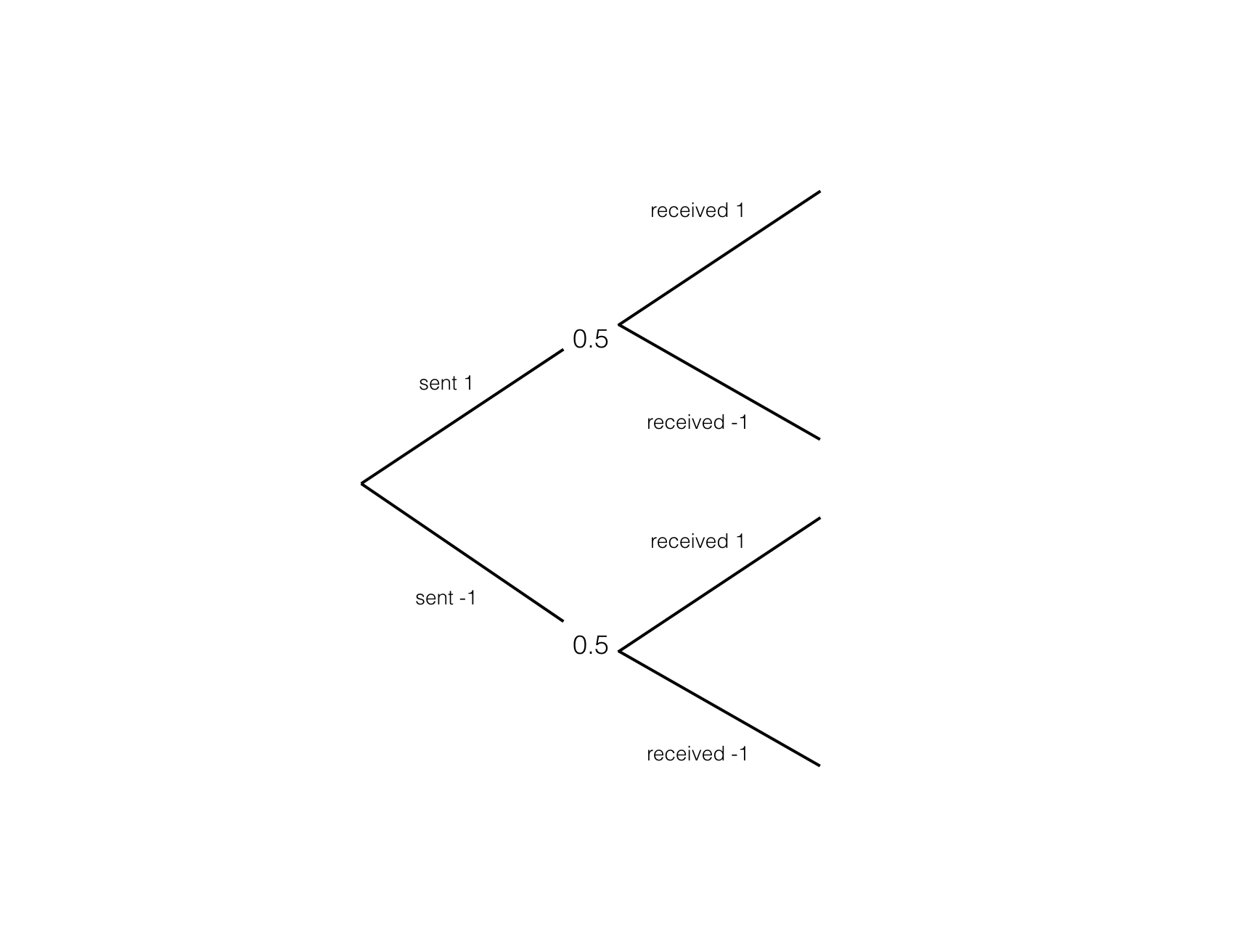The probability of transmitting a 0, the probability of transmitting a 1, the mean value for 0 transmissions, and the mean value for 1 transmissions:

In :
p0 = 0.5
p1 = 0.5
mu0 = -1.
mu1 = 1.


For a particular threshold, find the z-values:

We can find the z-values for each conditional probability:

\begin{align} Z_{+0.5} &= \frac{0.5 - \mu_{-1}}{\sigma_{-1}} \end{align}
\begin{align} Z_{-0.5} &= \frac{-0.5 - \mu_{1}}{\sigma_{1}}\\ \end{align}
In :
def getZvals(sigma):
return (thresh-mu0)/sigma, (-thresh-mu1)/sigma


We can plot the z-values on the normalized Gaussian distribution to see the sections that we'll be integrating:

In :
def showZVals(mu=0, Gc=0):
sigma = getNoiseSTD(signal, Gc=Gc)
z1, z2 = getZvals(sigma)
x = numpy.linspace(-5,5,1000)
curve = (1./(sigma*numpy.sqrt(2*numpy.pi)))*numpy.exp((-(x-mu)**2.)/(2*sigma**2.))
spl = InterpolatedUnivariateSpline(x, curve)
y1 = numpy.linspace(0, spl(z1), 1000)
y2 = numpy.linspace(0, spl(z2), 1000)
plt.plot(x, curve)
plt.plot(numpy.ones(len(y1))*z1, y1, 'r--', label='z-vals')
plt.plot(numpy.ones(len(y1))*z2, y2, 'r--')
plt.legend(loc='best')
plt.title('Z-Distribuion')
plt.show()
print('Z1: '+str(z1))
print('Z2: '+str(z2))
showZVals(Gc=0)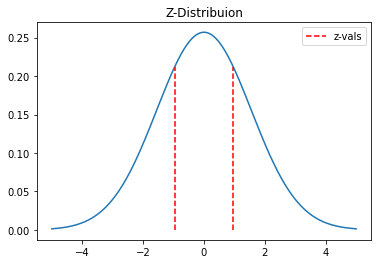Z1: 0.9664581242862053
Z2: -0.9664581242862053


Write a function to integrate for the conditional probabilities:

In :
def derivs(y, t, sigma, mu):
dydt = [(1./(sigma*numpy.sqrt(2*numpy.pi)))*numpy.exp((-(t-mu)**2.)/(2*sigma**2.))]
return dydt

def getIntegral(zval, Gc=0):
t = numpy.linspace(-9*getNoiseSTD(signal, Gc=Gc), zval, 5000)
y0 = [0.]
sigma = getNoiseSTD(signal, Gc=Gc)
sol = odeint(derivs, y0, t, args=(sigma, 0.))
return sol[:,0]


Make sure this is correct by verifying that the curve is normalized:

In :
def testIntegral(Gc=0):
test=getIntegral(10)
sigma = getNoiseSTD(signal, Gc=Gc)
plt.plot(numpy.linspace(-9*sigma, 9*sigma, 5000), test)
plt.plot(numpy.linspace(-9*sigma, 9*sigma, 5000), numpy.ones(len(test)), 'r--')
plt.xlim([-10, 10])
plt.show()
testIntegral()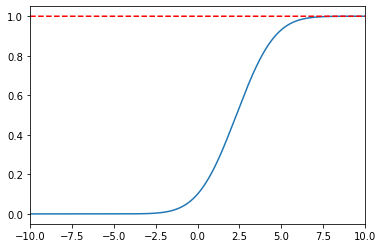Use the above to write a function for the conditional probability:

In :
def conditionalProb(sigma, Gc=0):
z = min(getZvals(sigma))
prob = getIntegral(z, Gc=Gc)[-1]
return prob


We can now find the total probability of a bit error:

In :
def bitErrorRate(Gc, signal=signal):
sigma = getNoiseSTD(signal, Gc=Gc)
perr = conditionalProb(sigma, Gc=Gc)
return p0*perr + p1*perr
bitErrorRate(Gc=0)

Out:
0.2667428705574693

This gives a total probability of bit error of:

\begin{align} p(error) &= 26.7\text{ percent} \end{align}

This indicates that 26.7 percent of transmitted bits will be corrupted. This is an intolerable amount of bit error, which can be improved by adding coding gain to the transmission.

#### How much Coding Gain is Required?¶

Let's plot the bit error rate as a function of coding gain:

In :
def BERvsGc():
Gcs = numpy.linspace(0, 16, 100)
BERs = numpy.zeros(len(Gcs))
for i in range(len(Gcs)):
BERs[i] = bitErrorRate(Gc=Gcs[i])
plt.plot(Gcs, BERs*100, label='bit error rate')
plt.xlabel('Gc (dBm)')
plt.ylabel('Bit Error Rate (percent)')
plt.title('Bit Error Rate vs. Coding Gain')
plt.yscale('log')
plt.legend(loc='best')
plt.show()
BERvsGc()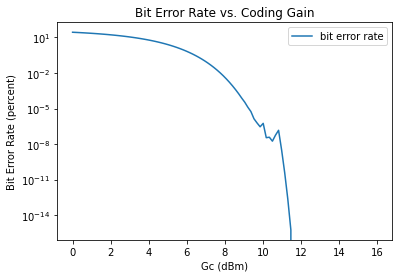## The Shannon-Hartley Theorem (Quick Sidestep)¶

#### Introducing the Theorem¶

The noisy channel coding theorem states that for any given degree of noise contamination of a communication channel, discrete data can be communicated error-free up to a computable maximum rate through the channel. If we apply this to the case of continuous-time analog communications in the presence of Gaussian noise, we arrive at the Shannon-Hartley Theorem.

\begin{align} C = B \text{log}_2\left(1 + \frac{S}{N}\right) \end{align}

This theorem establishes the channel capacity for the communication link, which is a bound on the maximum amount of error-free information that can be transmitted per unit time with a specified bandwidth. It is assumed that the signal power is bounded, and that the Gaussian noise is characterized by a known power or power spectral density.

\begin{align} C &= \text{channel capacity (bits/second)} \end{align}\begin{align} B &= \text{bandwidth of the channel (Hz)}\\ \end{align}\begin{align} S &= \text{average received signal power over the bandwidth (watts)}\\ \end{align}\begin{align} N &= \text{average power of the noise and interference over the bandwidth (watts)}\\ \end{align}\begin{align} \frac{S}{N} &= \text{signal to noise ratio} \end{align}

#### Applied to the Sprite¶

If we substitute the Sprite's bandwidth and SNR into the above equation, we find:

In :
def getShannon():
return B*numpy.log2(1+getSNRRatio())
getShannon()

Out:
25046.702519278784
\begin{align} \boxed{C = 25 kbps} \end{align}

This means that the maximum amount of error-free information that can be communicated via this noisy channel is 25 kilobits per second. If a line rate of less than 25 kbps is used, there exists a coding technique (involving error correction) which allows the probability of error at the receiver to be made arbitrarily small. The theorem tells us nothing about what this coding technique is, however.

## Improving the Signal to Noise Ratio¶

A matched filter is obtained by correlating a known signal (or template) with an unknown signal to detect the presence of the template in the unknown signal. A matched filter is the optimal linear filter for maximizing the signal to noise ratio in the presence of additive stochastic noise. A proof of this is given below (from Wikipedia):

Consider the observed signal $x$, which is composed of the desirable signal $s$ and additive noise $\nu$:

\begin{align} x = s + \nu \end{align}

We seek a filter $h$ of the form shown below that will maximize the signal to noise ratio.

\begin{align} y[n] &= \sum_{k=-\infty}^{\infty}h[n-k]x[k] \end{align}

Let us define the covariance matrix for the noise (which is Hermitian):

\begin{align} R_{\nu} &= E\left[\nu \nu^{H}\right]\\ \end{align}

Consider the output, $y$, which is the inner product of our filter and the received signal:

\begin{align} y &= \sum_{k=-\infty}^{\infty}h^{*}[k]x[k]\\ &= h^{H}x\\ &= h^{H}s + h^{H}\nu\\ &= y_s + y_{\nu} \end{align}

To be clear, we're considering some linear filter $h$. The output of our filter is the inner product of $h$ and the received signal, which is composed of the desired signal $s$ and the noise $\nu$. In the above equation, we consider the filter output from the signal, $y_s$, and the filter output from the noise, $y_{\nu}$. To maximize the signal to noise ratio, we want to maximize the ratio below:

\begin{align} SNR &= \frac{|y_s|^2}{E\left|[y_{\nu}|\right]^2}\\ &= \frac{|h^{H}s|^{2}}{E\left[|h^{H}\nu|^{2}\right]} \end{align}

Expanding the denominator:

\begin{align} E\left[|h^{H}\nu|^{2}\right] &= E\left[\left(h^{H}\nu\right)\left(h^{H}\nu\right)^{H}\right]\\ &= E\left[\left(h^{H}\nu\right)\left(\nu^{H}h\right)\right]\\ &= h^HE\left[\nu \nu^{H}\right]h\\ &= h^HR_{\nu} h \end{align}

Rewriting the SNR:

\begin{align} SNR &= \frac{|h^{H}s|^{2}}{h^H R_{\nu} h} \end{align}

We now pull a trick, rewriting the numerator to lead ourselves to the Cauchy-Schwartz inequality:

\begin{align} SNR &= \frac{|h^{H}s|^{2}}{h^H R_{\nu} h}\\ &= \frac{|h^H R_{\nu}^{\frac{1}{2}}R_{\nu}^{-\frac{1}{2}}s|^{2}}{h^H R_{\nu}^{\frac{1}{2}}R_{\nu}^{\frac{1}{2}} h}\\ &= \frac{\left|\left(R_{\nu}^{\frac{1}{2}}h\right)^H\left(R_{\nu}^{-\frac{1}{2}}s\right)\right|^2}{\left(R_{\nu}^{\frac{1}{2}}h\right)^H\left(R_{\nu}^{\frac{1}{2}}h\right)} \end{align}

We want to find an upper limit on the above expression. First, we recognize a form of the Cauchy-Schwartz Inequality:

\begin{align} \left| a^H b \right|^2 \leq \left(a^Ha\right)\left(b^H b\right) \end{align}

Applying this to the above expression:

\begin{align} \frac{\left|\left(R_{\nu}^{\frac{1}{2}}h\right)^H\left(R_{\nu}^{-\frac{1}{2}}s\right)\right|^2}{\left(R_{\nu}^{\frac{1}{2}}h\right)^H\left(R_{\nu}^{\frac{1}{2}}h\right)} \leq \frac{\left(R_{\nu}^{\frac{1}{2}}h \right)^H\left(R_{\nu}^{\frac{1}{2}}h \right)\left(R_{\nu}^{\frac{-1}{2}}s \right)^H\left(R_{\nu}^{\frac{-1}{2}}s \right)}{\left(R_{\nu}^{\frac{1}{2}}h\right)^H\left(R_{\nu}^{\frac{1}{2}}h\right)} \end{align}

Simplifying the term on the right:

\begin{align} \frac{\left|\left(R_{\nu}^{\frac{1}{2}}h\right)^H\left(R_{\nu}^{-\frac{1}{2}}s\right)\right|^2}{\left(R_{\nu}^{\frac{1}{2}}h\right)^H\left(R_{\nu}^{\frac{1}{2}}h\right)} \leq s^H R_{\nu}^{-1}s \end{align}

This limit is achieved when:

\begin{align} R_{\nu}^{\frac{1}{2}}h &= \alpha R_{\nu}^{-\frac{1}{2}}s \end{align}

Yielding the optimal filter:

\begin{align} \boxed{h = \alpha R_{v}^{-1}s} \end{align}

which makes sense intuitively. The linear filter is essentially a sliding dot product, and the above expression states that the SNR is best when the filter (the signal you're looking for) is parallel to the desired signal. One can choose $\alpha$ in accordance to the desired power of the filter output in response to noise (often unity).

\begin{align} 1 &= E\left[|h^{H}\nu\right|^2]\\ &=E\left[|s^H R_{\nu}^{-1}\alpha \nu|^2\right]\\ &= E\left[ \left(s^H R_{\nu}^{-1}\alpha \nu \right) \left(s^H R_{\nu}^{-1}\alpha \nu \right)^H\right]\\ &= s^H R_{\nu}^{-1}\alpha E\left[\nu \nu^H\right] \alpha R_{\nu}^{-1} s\\ &= s^H R_{\nu}^{-1}\alpha R_{\nu} \alpha R_{\nu}^{-1} s\\ &= \alpha^2 s^H R_{\nu}^{-1}s \end{align}

Solving for $\alpha$:

\begin{align} \alpha &= \frac{1}{\sqrt{s^H R_{\nu}^{-1} s}} \end{align}

which yields the normalized filter:

\begin{align} \boxed{h = \frac{R_{\nu}^{-1}}{\sqrt{s^H R_{\nu}^{-1} s}}s} \end{align}

## SNR and Coding Gain¶

Let's consider this equation from above one more time:

\begin{align} \frac{\left|\left(R_{\nu}^{\frac{1}{2}}h\right)^H\left(R_{\nu}^{-\frac{1}{2}}s\right)\right|^2}{\left(R_{\nu}^{\frac{1}{2}}h\right)^H\left(R_{\nu}^{\frac{1}{2}}h\right)} \leq s^H R_{\nu}^{-1}s \end{align}

The term on the right places an upper bound on the signal to noise ratio in terms of the noise covariance (something we have no control over) and the desired signal (something we do have control over). We are sending digital signals composed of 1's and 0's (converted to 1's and -1's for convenience). Let's consider how the bound on SNR changes with signal length:

###### Signal Length 1 (in $P_R=1$ Units)¶
\begin{align} SNR &\leq \begin{bmatrix} 1 \end{bmatrix} \begin{bmatrix} \sigma_{n}^{-2} \end{bmatrix} \begin{bmatrix} 1 \end{bmatrix}\\ &\leq 1\sigma_{n}^{-2} \end{align}
###### Signal Length 2¶
\begin{align} SNR &\leq \begin{bmatrix} 1 & 1 \end{bmatrix} \begin{bmatrix} \sigma_{n}^{-2} & 0\\ 0 & \sigma_{n}^{-2} \end{bmatrix} \begin{bmatrix} 1 \\ 1 \end{bmatrix}\\ &\leq 2\sigma_{n}^{-2} \end{align}
###### Signal Length N¶
\begin{align} SNR &\leq \begin{bmatrix} 1 & 1 & \cdots & 1 \end{bmatrix} \begin{bmatrix} \sigma_{n}^{-2} & 0 & 0 & 0 & \cdots\\ 0 & \sigma_{n}^{-2} &0 & 0 & \cdots\\ \vdots & \ddots & \ddots & 0 & \cdots\\ 0 & 0 & 0 & 0 & \sigma_{n}^{-2} \end{bmatrix} \begin{bmatrix} 1 \\ \vdots \\ 1 \end{bmatrix}\\ &\leq N\sigma_{n}^{-2} \end{align}
###### What this means¶

For a signal of length 1, the SNR is given by:

\begin{align} SNR &= \frac{P_R}{P_n}\\ &= \frac{1}{\sigma_n^2}\text{ (for $P_R=1$ units)} \end{align}

For a signal of length $N$:

\begin{align} SNR &= N\left(\frac{1}{\sigma_n^2}\right)\text{ (for $P_R=1$ units)} \end{align}

In dBm:

\begin{align} SNR &= 10\cdot \text{log}_{10}\left[N\left(\frac{1}{\sigma_{n}^2}\right)\right]\\ &= 10\cdot \text{log}_{10}\left[N\right] + 10\cdot \text{log}_{10}\left[\frac{1}{\sigma_{n}^2}\right]\\ &= 10\cdot \text{log}_{10}\left[N\right] + \left(P_R - P_n\right)\\ &= \left(10\cdot \text{log}_{10}\left[N\right] + P_R\right) - P_n \end{align}

With matched filtering, we have effectively increased the gain of the transmitted signal. The gain is related to the length of the filter as shown above. To be clear:

\begin{align} \boxed{G_c = 10\cdot \text{log}_{10}[N]} \end{align}

where $N$ is the length of the code. By adding 10 dBm of coding gain, we can see that the conditional probabilities that lead to bit error become far smaller, and the z-values increase in magnitude:

In :
drawHistos(Gc=10*numpy.log10(16))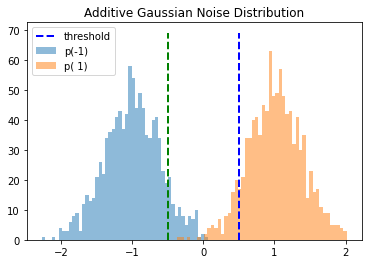In :
showZVals(Gc=10*numpy.log10(16))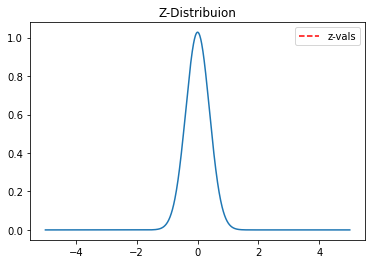Z1: 3.8658324971448224
Z2: -3.8658324971448224


## An Example¶

We want to transmit the message 'Greetings Earthlings.' Begin by converting this string to ASCII, and then to binary:

In :
signal = createSignal('Greetings Earthlings')
print((signal+1)/2)
plt.plot(signal)
plt.ylim([-1.5, 1.5])
plt.title('Message in Binary')
plt.show()

[0. 1. 0. 0. 0. 1. 1. 1. 0. 1. 1. 1. 0. 0. 1. 0. 0. 1. 1. 0. 0. 1. 0. 1.
0. 1. 1. 0. 0. 1. 0. 1. 0. 1. 1. 1. 0. 1. 0. 0. 0. 1. 1. 0. 1. 0. 0. 1.
0. 1. 1. 0. 1. 1. 1. 0. 0. 1. 1. 0. 0. 1. 1. 1. 0. 1. 1. 1. 0. 0. 1. 1.
0. 0. 1. 0. 0. 0. 0. 0. 0. 1. 0. 0. 0. 1. 0. 1. 0. 1. 1. 0. 0. 0. 0. 1.
0. 1. 1. 1. 0. 0. 1. 0. 0. 1. 1. 1. 0. 1. 0. 0. 0. 1. 1. 0. 1. 0. 0. 0.
0. 1. 1. 0. 1. 1. 0. 0. 0. 1. 1. 0. 1. 0. 0. 1. 0. 1. 1. 0. 1. 1. 1. 0.
0. 1. 1. 0. 0. 1. 1. 1. 0. 1. 1. 1. 0. 0. 1. 1.]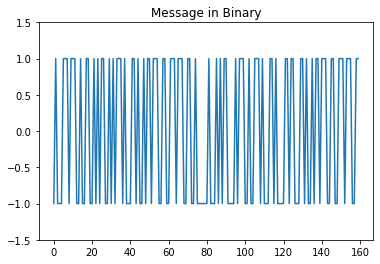Let's consider a matched filter of length 16, which will add $10\cdot \text{log}_{10}(16) \approx 12$dB of gain. We'll use the codes shown below (justified in a later section):

In :
code0 = numpy.array([numpy.ones(32)])
code1 = -1*numpy.array([numpy.ones(32)])


Write a function that creates a message, encodes it, and noisifies it:

In :
def encode(signal, code0, code1):
encoded_message = numpy.array([[]])
for i in signal:
if i==1:
encoded_message = numpy.hstack((encoded_message, code1))
else:
encoded_message = numpy.hstack((encoded_message, code0))
encoded_message = encoded_message
return encoded_message


Write a function to observe the encoded signal:

In :
def showEncoded():
encoded_message = encode(signal, code0, code1)
sigma = getNoiseSTD(encoded_message, Gc=0)
noise = makeNoise(sigma, encoded_message)
plt.plot(encoded_message+noise, label='encoded signal+noise');
plt.plot(encoded_message, 'r', lw=2, label='encoded signal')
plt.xlabel('Sample Number')
plt.legend(loc='best')
plt.show()
showEncoded()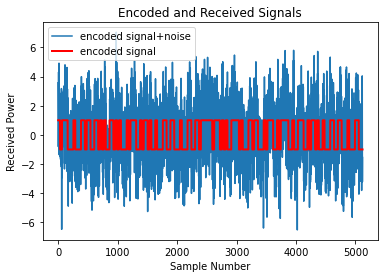Write a function to decode the noisy message:

\begin{align} \boxed{h = \frac{R_{\nu}^{-1}}{\sqrt{s^H R_{\nu}^{-1} s}}s} \end{align}
In :
def decode(encoded_noisy_message, code0, code1):
zero_dot_products = []
one_dot_products = []

chiplength = len(code0)
messagelength = len(encoded_noisy_message)

sigma = getNoiseSTD(signal)
scale = 1./(sigma**2. * numpy.sqrt((1./sigma**2.)*chiplength))

for i in range(0, messagelength - chiplength):
zdot = numpy.dot(numpy.array([encoded_noisy_message[i:i+chiplength]]), scale*code0.T)
odot = numpy.dot(numpy.array([encoded_noisy_message[i:i+chiplength]]), scale*code1.T)
zero_dot_products.extend([zdot])
one_dot_products.extend([odot])
return numpy.array(one_dot_products), numpy.array(zero_dot_products)


Write a function that takes a decoded message and finds the difference in the dot products:

In :
def testDecode():
encoded_message = encode(signal, code0, code1)

messagelen = len(encoded_message)
chiplen = len(code0)

sigma = getNoiseSTD(encoded_message, Gc=0)
noise = makeNoise(sigma, encoded_message)
encoded_noisy_message = encoded_message+noise

decoded1, decoded0 = decode(encoded_noisy_message, code0, code1)
difference = decoded1 - decoded0

plt.plot(decoded1 - decoded0, label='Decoded Message')
plt.grid('on')
plt.legend(loc='best')
plt.title('Raw and Filtered Signal')
plt.show()
return difference
decoded_message = testDecode();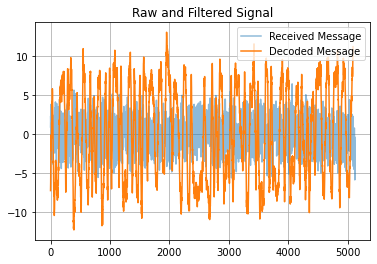Write a soft decoder to recover the transmitted message:

In :
def softDecode(filtered_message, code0, code1):
message = []
messagestr = ''
messagelen = len(filtered_message)
chiplen = len(code0)
for i in numpy.arange(0, messagelen-1, chiplen):
message.extend([int(filtered_message[i]/abs(filtered_message[i]))])
message.extend([int(filtered_message[-1]/abs(filtered_message[-1]))])
for i in message:
messagestr += str(int((i+1)/2))
return numpy.array(message), messagestr


Write a function for converting back to ASCII --> characters.

In :
def decodeCharacters(data):
message = ''
counter = 0
while counter < (len(data) - len(data)%8):
message+=chr(int(data[counter:counter+8],2))
counter+=8
return message


Decode, and show results:

In :
soft1, soft2 = softDecode(decoded_message, code0, code1)
print(soft2)
print('\n')
print('Difference between Sent and Received Messages:')
diff = soft1 - signal
print(diff)
print('\n')
print('Number of Errors:')
print(str(numpy.count_nonzero(diff))+'/'+str(len(signal))+' bits')
print('\n')
print('Received Message (converted back to characters)')
print(decodeCharacters(soft2))

Received Message:
0100011101110010011001010110010101110100011010010110111001100111011100110010000001000101011000010111001001110100011010000110110001101001011011100110011101110011

Difference between Sent and Received Messages:
[0. 0. 0. 0. 0. 0. 0. 0. 0. 0. 0. 0. 0. 0. 0. 0. 0. 0. 0. 0. 0. 0. 0. 0.
0. 0. 0. 0. 0. 0. 0. 0. 0. 0. 0. 0. 0. 0. 0. 0. 0. 0. 0. 0. 0. 0. 0. 0.
0. 0. 0. 0. 0. 0. 0. 0. 0. 0. 0. 0. 0. 0. 0. 0. 0. 0. 0. 0. 0. 0. 0. 0.
0. 0. 0. 0. 0. 0. 0. 0. 0. 0. 0. 0. 0. 0. 0. 0. 0. 0. 0. 0. 0. 0. 0. 0.
0. 0. 0. 0. 0. 0. 0. 0. 0. 0. 0. 0. 0. 0. 0. 0. 0. 0. 0. 0. 0. 0. 0. 0.
0. 0. 0. 0. 0. 0. 0. 0. 0. 0. 0. 0. 0. 0. 0. 0. 0. 0. 0. 0. 0. 0. 0. 0.
0. 0. 0. 0. 0. 0. 0. 0. 0. 0. 0. 0. 0. 0. 0. 0.]

Number of Errors:
0/160 bits

Received Message (converted back to characters)
Greetings Earthlings


## Bayesian Analysis of PRN Codes¶

Suppose our PRN codes are of length N. As the raw binary stream comes in, we can dot each N bits in the stream with each of the PRN codes. Because all of the codes are orthogonal, a perfect transmission of a particular PRN code will yield a dot product of N with the correct PRN, and a dot product of zero with all other PRN's. As shown in the previous sections, however, the bit error rate for individual bits is quite high for the case of the Sprite. This means that a transmission will have some degree of corruption, and will likely have a dot product $<N$ with the correct PRN code and a dot product $>0$ with some of the incorrect PRN codes. Furthermore, it's not always the case that the Sprite is transmitting information. Random noise will have some power in some of the PRN codes (and, on rare occasion, it may have a great deal of power along a particular PRN code).

All of the PRN codes are orthogonal. If they are of length N, then we can construct N orthogonal codes using Hadamard recursion. If we normalize all of these PRN codes, we can think of them as the basis vectors for a frame that is rotated relative to the frame of the incoming data. By normalizing all of the PRN codes, stacking them in columns, and then combining those columns into a matrix, we form a rotation matrix between the data frame and the frame for which the PRN codes are simply the columns of an identity matrix. This is useful because it makes it very easy to see how much power a particular string of bits has in each PRN code.

A string of bits may have power in a particular PRN code because:

1. The Sprite transitted that PRN code
2. The Sprite transmitted a different PRN code, but a bit error put some power into that particular PRN code
3. The Sprite did not transmit anything, and random noise put some power into that particular PRN code

Via a nonlinear transformation, we can transform each of these vectors (now represented in the PRN frame) such that their magnitude in each direction (along each PRN code) is precisely the probability that a Sprite transmitted that PRN code.

This is useful because it means the output of the filter (which is now nonlinear and time-varying) is a meaningful quantity. Rather than looking for spikes associated with dot products, we can say for every string of $N$ bits precisely how confident we are that that string of bits came from a particular Sprite vs. any other Sprite vs. noise.

In :
def hadamardRecursion(n):
if n == 1:
return numpy.array([[1.]])
else:
return numpy.vstack((top, bottom))


### $p(j|PRNx)$¶

Consider the case where PRNx, of length $N$, is being transmitted. Suppose that the value of the dot product associated with PRNx is $j$. Find the conditional probability $p(PRNx|j)$.

In order to get the value $j$, $n$ bit flips are required, where $n$ is:

\begin{align} n = \frac{N-j}{2} \end{align}

In order to get $j$ for the dot product, any $n$ of the $N$ bits must flip. It doesn't matter which $n$. The number of ways that one can choose $n$ bits from the $N$ bits is given by:

\begin{align} k = \frac{N!}{n!(N-n)!} \end{align}

All of these potential solutions have the same probability:

\begin{align} p(k_{i}) = B^n\left(1-B\right)^{N-n} \end{align}

Where $B$ is the bit error rate. Since any of these possibilities will do, to get the probability of any of them happening we sum the probability of each of them occuring:

\begin{align} p\left(j|PRNx\right) &= KB^n\left(1-B\right)^{N-n}\\ &= \left[\frac{N!}{n!\left(N-n\right)!}\right]B^n\left(1-B\right)^{N-n}\\ &= \left[\frac{N!}{\left(\frac{N-j}{2}\right)!\left(N-\frac{N-j}{2}\right)!}\right]B^{\left[\frac{N-j}{2}\right]}\left(1-B\right)^{\left[N-\frac{N-j}{2}\right]} \end{align}
In :
def pJgivenPRNx(N, j, B):
first_term = numpy.math.factorial(N)/(numpy.math.factorial(int((N-j)/2.))*numpy.math.factorial(int(N - ((N-j)/2.))))
second_term = B**((N-j)/2.)
third_term = (1-B)**(N - ((N-j)/2.))
return first_term*second_term*third_term


Write a function to visualize this conditional probability for all possible dot products $j$ as the bit error rate is varies between 0 and 1:

In :
 def testpJgivenPRNx(N):
berr = numpy.arange(0., .5025, 0.025)
for j in berr:
test = []
for i in numpy.arange(-N, N+1):
test.extend([pJgivenPRNx(N, i, j)])
plt.plot(numpy.arange(-N, N+1), test, '-', label='Bit Error: '+str(j))
plt.xlim([-N,N])
plt.ylabel('$p(j|PRNx)$')
plt.title('$p(j|PRNx)$')